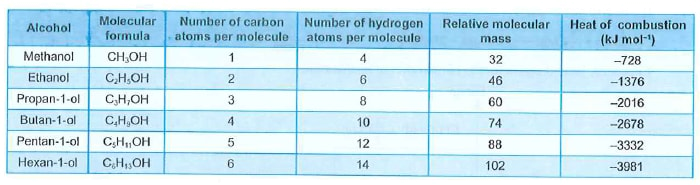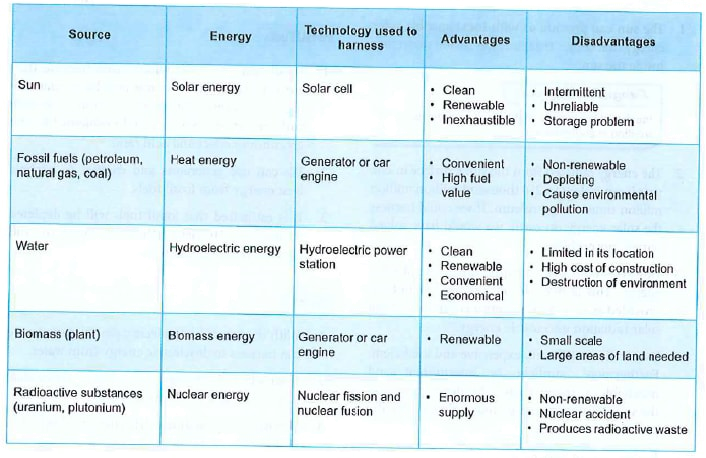##### Heat of Combustion

1. A fuel is a chemical substance that burns in oxygen to produce heat energy.

2. Combustion is a chemical reaction between a fuel and oxygen to release heat. Combustion is always an exothermic reaction.

3. The heat of combustion is the heat produced when one mole of a substance is completely burnt in oxygen under standard conditions.

4. The substances can be elements or compounds.

(a) Combustion of elements

$$C(s)+O_2(g)\rightarrow CO_2(g)$$  $$\triangle H=-394 kJ$$When 1 mole of carbon burns completely in oxygen to form carbon dioxide, 394 kJ of heat is released. The heat of combustion of carbon is -394 kJ $$mol^-1$$. The energy level diagram for the combustion of carbon is as shown below.

(b) Combustion of compounds

$$CH_4(g)+2O_2(g)\rightarrow CO_2(g)+2H_2O(l)$$  $$\triangle H=-890 kJ$$When 1 mole of methane burns completely in oxygen to form carbon dipxide and water, 890 kJ of heat is released. The heat of combustion of methane is -890 kJ $$mol^-1$$. The energy level diagram for the combustion of methane is as shown in Figure 4.29.

5. Excess oxygen is necessary during combustion to make sure that the combustion is complete.

6. Incomplete combustion due to insufficient oxygen may result in different products being formed, and thus the heat given out would be different.

(a) In excess oxygen, 1 mole of carbon burns completely to form carbon dioxide and
produce 394 kJ of heat.

$$C(s)+O_2(g)\rightarrow CO_2(g)$$  $$\triangle H=-394 kJ$$

(b) In limited supply of oxygen, 1 mole of carbon burns to form carbon monoxide and
produce 108 kJ of heat.

$$C(s)+\frac{1}{2}O_2(g)\rightarrow CO(g)$$  $$\triangle H=-108 kJ$$

Note: The heat of combustion of carbon is $$-394\ kJ\ mol^{-1}$$ and not $$-108\ kJ\ mol^{-1}$$.

7. When writing thermochemical equation, we can use fractions to represent the number of moles of oxygen required so that the number of moles of the substance is always 1 mole.

8. Different fuels have different values of heat of combustion.

9. The value of heat of combustion is an important factor for us to decide what fuel to use for a certain purpose.

Table 4.15 Values of heat of combustion for some fuels

 Fuel Use Equation during combustion Heat of combustion, $$\triangle H (kJ\ mol^{-1})$$ Methane Heating, cooking $$CH_4(g)+2O_2(g)\rightarrow CO_2(g)+2H_2O(l)$$ -890 Hydrogen Rocket fuel $$H_2(g)+\frac{1}{2}O_2(g)\rightarrow H_2O(l)$$ -286 Glucose Body fuel $$C_6H_{12}O_6(s)+6O_2(g)\rightarrow 6CO_2(g)+6H_2O(l)$$ -2803 Butane Cooking $$C_4H_{10}(g)+\frac{13}{2}O_2(g)\rightarrow 4CO_2(g)+5H_2O(l)$$ -2877

Determining the heat of combustion

1. The heat of combustion of a fuel can be determined accurately by using a bomb calorimeter.2. An insulated container is filled with a known quantity of water. A small sample of known mass in the steel bomb is ignited electrically and burnt completely. The heat given out in the reaction is determined from the temperature rise in the water that surrounds the bomb.

3. The bomb calorimeter is specifically designed to minimise heat loss to the surroundings.

4. In the laboratory, the heat of combustion of a fuel can be determined as follows.

(a) A liquid fuel is burnt is excess oxygen.

(b) The mass and the number of moles of the fuel are determined.

(c) The heat produced is used to heat a known mass of water.

(d) The increase in the temperature of the water is measured.

(e) The heat absorbed by the water is calculated using the formula $$H=mc\theta$$.

(f) The heat given out by the fuel is calculated. We assume that all the heat given out
during the combustion of the fuel is absorbed by the water.

Heat given out during combustion of the fuel = heat absorbed by the water

(g) The heat of combustion (heat given out by 1 mole of the fuel) is calculated.

Heat of combustion of various alcohols

1. One example of liquid fuel is alcohol. Different members of the alcohol family have different heat of combustion. The table below shows the heat of combustion of some alcohols.

Table 4.17 Heat of combustion of some alcohols2. Table 4.17 shows that the heat of combustion of alcohol increases as the

(a)  number of carbon atoms per molecule increases

(b) number of hydrogen atoms per molecule increases

(c) relative molecular mass increases

3. During the combustion of alcohol:

(a) Carbon atom is burnt to form carbon dioxide.

$$[C]+O_2(g)\rightarrow CO_2(g)+$$ heat

(b) Hydrogen atom is burnt to form water.

$$2[H]+\frac{1}{2}O_2(g)\rightarrow H_2O(l)+$$ heat

(c) Both the reactions are exothermic. Therefore, more heat is produced when more carbon
and hydrogen atoms are burnt.

(d) Relative molecular mass is proportional to the number of carbon and hydrogen atoms
per molecule. Thus, the heat of combustion of alcohol increases as the relative
molecular mass increases.

4. The difference in the heat of combustion of successive members is almost the same, that is, about 650 kJ.

(a) Successive members of the homologous series of alcohols differ from each other by
a $$-CH_2$$ group.

(b) The constant increase in the heat of combustion of the successive members of alcohol
is due to the extra heat given out by the extra one carbon atom and two hydrogen
atoms in the $$-CH_2$$ group.

Table 4.18 Difference in heat of combustion of successive members

 Pair of alcohols Difference in the heat of combustion (Kj) Methanol and ethanol 1376 – 728 = 648 Ethanol and propan-1-ol 2016 – 1376 = 640 Propan-1-ol and butan-1-ol 2678 – 2016 = 662 Butan-1-ol and pentan-1-ol 3332 – 2678 = 654 Pentan-1-ol and hexan-1-ol 3981 – 3332 = 649

5. When the heat of combustion is plotted against the number of carbon atoms per alcohol molecule, the graph in Figure 4.33 is obtained.Fuel value

1. Fuels are substances that can normally burn readily in air or oxygen to produce large quantity of heat.

2. Examples of some common fuels are petroleum, natural gas and coal. These are called fossil fuels.

3. Different types of fuels have different heats of combustion. Heat of combustion is expressed in kilojoules per mole.

4. In our daily life, it is not very practical to measure the quantity of a fuel in mole. We normally measure the quantity of a fuel by its mass.

5. The fuel value of a fuel is the amount of heat energy given out when one gram of the fuel is completely burnt in excess of oxygen.

6. The unit for fuel value is $$kJ\ g^{-1}$$.

7. Fuel value is also known as heat value.

8. A fuel with a high fuel value releases a lot of heat per gram when it burns.

9. Different types of fuels have different fuel values. Some fuels have very high fuel values but have low density, thus occupy a bigger volume. Others have high density but have low fuel values.

10. Some fuels can cause environmental pollution. There are fuels with high fuel values but are not very economical, not easily available or non-renewable.

11. The learning of thermochemistry helps us in the selection of a suitable fuel for a specific purpose.

12. The table below shows the fuel values of some food and common fuels.

Table 4.19 Fuel values of some food and common fuels

 Substance Fuel value $$(kJ\ g^{-1})$$ Apple 2 Egg 6 Bread 11 Wood 20 Coal 30 Butter 34 Gasoline 34 Kerosene 37 Natural gas 50 Hydrogen 143

13. The selection of a fuel to be used in industries depends on the following factors:

(a) Fuel value of the fuel

(b) Cost of the fuel

(c) Availability and sources of the fuel

(d) Effect of the fuel on the environment

(e) Technology used to harness the energy from the fuel

(f) Storage space needed to store the fuel

14. Fuels with the following qualities are normally chosen.

(a) High fuel value

(b) Cheap

(c) Easily available

(d) Do not pollute the environment

(e) Easily harnessed

(f) Require less storage space

The existence of various energy sources

We can find many sources of energy such as the sun, fossil fuels, water, biomass and radioactive substances on our earth.

The sun

1. The sun can provide us with in exhaustible solar energy that comes from nuclear fusion occurring inside the sun.

2. The energy radiated from the sun's surface in one year is equivalent to 250 thousand million million million tonnes of petroleum. If we could harness the solar energy on earth, we would have solved our energy problem.

3. However, we make very little direct use of solar energy. This is because technology has not yet provided an economical method of transforming solar radiation into usable energy.

4. Solar cells are still very expensive and inefficient. Furthermore, sunlight is intermittent and unreliable in many areas. The development in the storage of solar energy may help us solve the problem.

5. The knowledge of thermochemistry can help us store solar energy.

(a) Ionic hydrates such as hydrated sodium sulphate, $$Na_2SO_4.10H_2O$$ can be used to store
solar energy.

(b) When the hydrates are warmed by sunlight to over 32°C, the three moles of ions
dissolve in the ten moles of water in an endothermic process.

Hydrated sodium sulphate $$[Na_2SO_4.10H_2O(s)]$$ → aqueous sodium sulphate $$[Na_2SO_4(aq)]$$
$$\triangle H=+354\ kJ$$

(c) When cooled below 32°C  after sunset, the solution recrystallises, releasing the
absorbed energy.

Aqueous sodium sulphate $$[Na_2SO_4(aq)]$$ → Hydrated sodium sulphate $$[Na_2SO_4.10H_2O(s)]$$
$$\triangle H=-354\ kJ$$

Fossil fuels

1. Fossil fuels are most widely used because they are very convenient to use and have relatively high fuel values. But they are non-renewable and can cause environmental problems like the greenhouse effect and acid rain.

2. We can use generators and engines to harness heat energy from fossil fuels.

3. It is estimated that fossil fuels will be depleted in another 10 years. Thus, we need to find alternative energy sources.

Water

1. With the use of hydroelectric power stations, we can harness hydroelectric energy from water.

2. Hydroelectric energy is clean, renewable, convenient and economical to use.

3. Unfortunately, hydroelectric energy is limited in its location. It needs high cost of construction at the beginning stages and sometimes vast areas of land are needed, and thus might cause destruction of the surrounding environment.

Biomass

1. By using generators and engines we can harness biomass energy.

2. We can obtain biomass energy from either plants or droppings of animals. Palm oil, for example, is used to make biodiesel which can be used to power cars and generators.

3. Biomass energy, however, needs large areas of land to grow plants and may also cause environmental problems like the disposal of waste.

1. By using nuclear fission and nuclear fusion, we could obtain enormous supply of nuclear energy from radioactive substances like uranium and plutonium.

2. The heat given out during controlled nuclear fission and fusion can be used to generate electricity.

3. Nuclear energy is non-renewable and is very destructive if a nuclear accident occurs. Furthermore, we will face problems of disposing the nuclear wastes.

Table 4.20 Major sources of energy harnessed and their advantages and disadvantagesOther energy sources

1. Other energy sources that we can use are wind, tidal, geothermal, chemical fuel cells and hydrogen from the electrolysis of sea water.

2. Scientists and engineers are actively seeking new energy sources as the earth has only a limited supply of fossil fuels which will run out soon.

3. We must learn to use and conserve out finite energy resources more wisely and more efficiently.